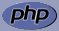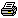www.PHPBuddy.comPHP Function Lookup:

 Categories
 PHP Quick Start PHP Installation PHP Articles PHP Scripts

 Top Rated Articles

 Site Related
 Submit Articles/Code Contact UsArticle Added on: April 15, 2002Overview: Introducing the if else construct, while loops and other control loops that will help add logic to your code.

Okay our task is to make our programs intelligent I mean let's learn how to add decision making logic in our code. PHP like all other good programming languages provides logic and looping using the if and while statements.

Introducing the IF construct
The if construct is one of the basic decision making construct it is also included in PHP. It allows for conditional execution of code fragments. PHP features an if structure that is similar to that of the 'C' language.

The syntax is of if else structure is ``` If (conditions) { // Code if condition is true } else { // Code if condition is false }```

Let me give you an example, let's assume that you and your friend are planning to go for a movie you need more than 20 bucks to go to the movie, if you have less than 20 bucks you can't go, if you would have written a programme the PHP equalent code would be something like

```<?php \$money = 15; if(\$money > 20) { echo "Hey we can go to the movie we have \$money bucks"; } else { echo "Oops we can't go to the movie we have only \$money bucks"; } ?>```

We have used the Comparison operator > Greater than above to check if \$money > 20

PHP supports many different comparison operators that allow us to add logic in our code.

The comparison operators are listed below:
```\$a == \$b \$a is equal to \$b \$a != \$b \$a is not equal to \$b \$a < \$b \$a is less than \$b \$a > \$b \$a is greater than \$b \$a <= \$b \$a is less than or equal to \$b \$a >= \$b \$a is greater than or equal to \$b```

Now we know how to add logic using if else and comparison operators to our code in the next part we will learn the basic's of looping.